# NCERT Solutions for Class 8 Maths Chapter 7 Cube and Cube Roots Ex 7.1

These NCERT Solutions for Class 8 Maths Chapter 7 Cube and Cube Roots Ex 7.1 Questions and Answers are prepared by our highly skilled subject experts.

## NCERT Solutions for Class 8 Maths Chapter 7 Cube and Cube Roots Exercise 7.1

Question 1.
Which of the following numbers are not perfect cubes?
(i) 216
(ii) 128
(iii) 1000
(iv) 100
(v) 46656
Solution:
(i) 216
216 = 23 × 33
Each prime factors appear in triplets, so 216 is a perfect cube.(ii) 128
128 = 23 × 23 × 2
2 remains after grouping in triplets, so 128 is not a perfect cube.(iii) 1000
1000 = 23 × 53
Each prime factor appears in triplets so 1000 is a perfect cube.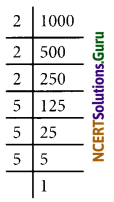(iv) 100
100 = 22 × 52
As we do not get any triplets, So 100 is not a perfect cube.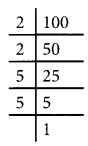(v) 46656
46656 = 23 × 23 × 33 × 33
Each prime factor appears in triplets, so 46656 is a perfect cube.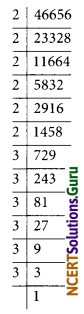Question 2.
Find the smallest number by which each of the following numbers must be multiplied to obtain a perfect cube.
(i) 243
(ii) 256
(iii) 72
(iv) 675
(v) 100
Solution:
(i) 243
243 = 33 × 32
On grouping the factors in triplets, the prime factor 3 does not appear in a group of three.
To make it a perfect cube, we need one more 3.
The number 243 should be multiplied by 3 to get a perfect cube.(ii) 256
256 = 23 × 23 × 22
On grouping the factors in triplets, the prime factor 2 does not appears in a group of three.
To make it a perfect cube, we need one more 2.
The number 256 should be multiplied by 2 to get a perfect cube.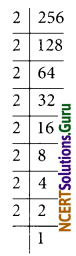(iii) 72
72 = 23 × 32
On grouping, the factors in triplets the prime factor 3 does not appear in a group of 3.
To make it a perfect cube, we multiply it by 3.
The number 72 should be multiplied by 3 to get a perfect cube.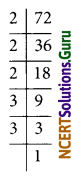(iv) 675
675 = 33 × 52
On grouping the factors in triplets, the prime factor 5 does not appear in a group of three.
To make it a perfect cube, we multiply it by 5.
The number 675 should be multiplied by 5 to get a perfect cube.(v) 100
100 = 22 × 52
On grouping the factors in triplets, the prime factors 2 and 5 do not appear in a group of three.
To make it a perfect cube we need one 2 and one 5 (2 × 5 = 10)
The number 100 should be multiplied by 10 to get a perfect cube.Question 3.
Find the smallest number by which each of the following numbers must be divided to obtain a perfect cube.
(i) 81
(ii) 128
(iii) 135
(iv) 192
(v) 704
Solution:
(i) 81
81 = 33 × 3
On grouping the factors in triplets, we find that three is no triplet of 3.
To make it a perfect cube, we divide it by 3.(ii) 128
128 = 23 × 23 × 2
On grouping the factors in triplets, we find that there is no triplet of 2.
To make it a perfect cube, we divide it by 2.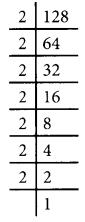(iii) 135
135 = 33 × 5
On grouping the factors in triplets, the prime factor 5 does not appear in a group of three.
To make it a perfect cube, 135 should be divided by 5.(iv) 192
192 = 23 × 23 × 3
On grouping the factors in triplets, the prime factor 3 does not appear in a group of three.
To make it a perfect cube, 192 should be divided by 3.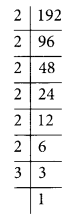(v) 704
704 = 23 × 23 × 11
On grouping the factors in triplets, the prime factor 11 does not appear in a group of three.
To make it a perfect cube, 704 should be divided by 11.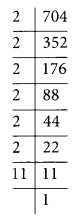Question 4.
Parikshit makes a cuboid of plasticine on sides 5 cm, 2 cm, 5 cm. How many such cuboids will he need to form a cube?
Solution:
Sides of the cuboid are 5 cm, 2 cm, and 5 cm
Volume of the cuboid = 5 cm × 2 cm × 5 cm
To form it as a cube, its dimension should be in the group of triplets.
Since there is only one 2 and only two 5 in the prime factorization.
We need 2 × 2 × 5 = 20 such cuboids to make a cube.

error: Content is protected !!